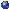## Events

##### Junior AnalysisJoseph S Jackson, : The non-linear Feynman-Kac formula
Friday, March 26, 2021, 11:00am - 12:00pm
There is a fundamental and very well-known connection between linear PDEs and SDEs. One manifestation of this connection is the Feynman-Kac formula, which provides a stochastic representation for solutions to a certain linear parabolic equation. After reviewing the Feynman-Kac formula, I will introduce a slightly less well-known bridge between PDEs and stochastic analysis, namely the non-linear Feynman-Kac formula. The non-linear Feynman-Kac formula, unlike its linear cousin, uses BSDEs instead of SDEs, and provides a stochastic representation of solutions to a class of semi-linear parabolic PDEs.